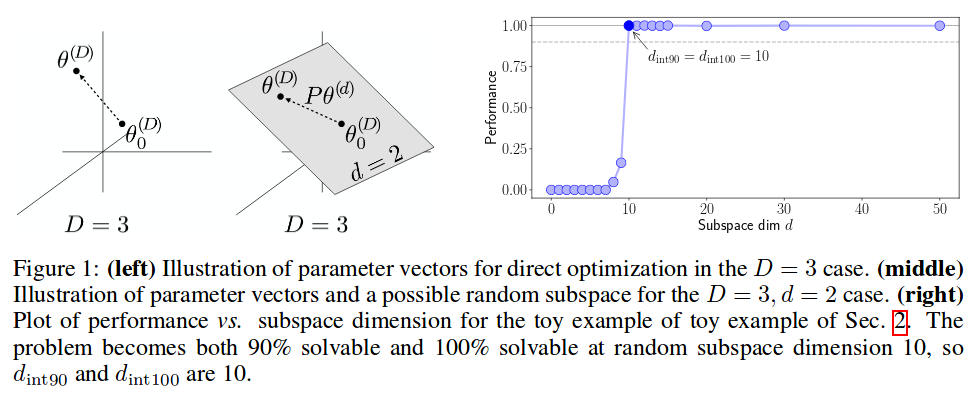In this paper, the authors propose a novel metric to describe the difficulty of solving a task for a family of networks architectures on a given dataset. This metric is called Intrinsic Dimension. For example, the intrinsic dimension of classifying MNIST digits using a MLP is 750. Adding more layers or widening the network does not change this metric.

This metric is obtained by optimizing network parameters along random subspaces, and finding the minimum dimension of a subspace that solves the task.

# Subspace optimization

Typically, when optimizing a MLP with a parameter vector $$\theta^{(D)}$$ of size $$D$$, we take steps on the full “native space” $$\theta^{(D)}$$. In “subspace optimization”, we sample $$d$$ < $$D$$ random directions in $$\mathbb{R}^D$$, and we optimize in the subspace $$\theta^{(d)}$$. Thus, we need a mapping from $$\theta^{(d)}$$ to $$\theta^{(D)}$$:

$\theta^{(D)} = \theta^{(D)}_0 + P\theta^{(d)}$

where $$P$$ is a $$D \times d$$ projection matrix, and $$\theta^{(D)}_0$$ is the initialization of the parameters. Below is a visual example for a two-layer MLP:Please take a moment to notice that we typically don’t have $$\theta^{(d)}$$ and $$P$$, and we take steps on $$\theta^{(D)}$$. However, here, we take steps on $$\theta^{(d)}$$. Also note that $$P$$ is not trainable; it is determined at the beginning of training.# Measuring the Intrinsic Dimension

1. Take a specific model architecture
2. Take a subspace dimension $$d$$ and sample many subspaces in it
3. Construct $$P$$ for each of these subspaces
4. Repeat steps 2 and 3 for many $$d$$
5. For each different $$P$$, train the model using this subspace
6. Plot a graph like Figure 1 (right) to determine the minimal $$d$$ where the task is considered solved.

$$d_{int100}$$ (“int” for intrinsic) is the $$d$$ for which the authors obtain the same performance as using the full native space. $$d_{int90}$$ is the $$d$$ for which the authors obtain 90% of the performance. For their experiments, they use $$d_{int90}$$ as the intrinsic dimension. See paper for a justification.